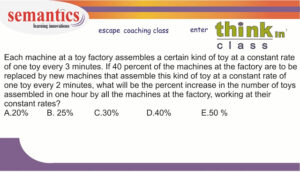# How to overcome GRE math anxiety?Do you have a non-math background?

Has it been a long time since you touched your math books?

Feeling math-anxious?

Do you have a non-math background?

Has it been a long time since you touched your math books?

Feeling math-anxious?

Many students ask me this question.

“Can I ace the GRE math, if I have a non-math background?”   Yes, you can.

Remember GRE tests you on math reasoning…

To overcome anxiety, You need to learn math concepts.+ You need to develop math skill-sets too.

Formula/theorems (Area of the circle, HCF and LCM of numbers…)  are the math concepts.

So what are the math skill-sets?

Definition of a skill-set: A skill-set is the combination of knowledge and abilities that you’ve developed through your life and work. You use these skills-sets to tackle problems.

We, at semantics, have developed modules, which can hone a students math and verbal skill sets.

Let me take you through one math skill-set.

Problem Decomposition

Definition: Decomposition is  the process of breaking a problem down into smaller parts to make it easier to tackle.

If you have a non- math background, you might find it difficult to comprehend word problems.

One way to analyze word problems is – break problems into meaning-full chunks.

You need to

1. break the problem into  2 or more segments.
2. Analyse each segment.
3. Infer data (if necessary)
4. Diagram information…

Only after all this, you write equations and solve..

Let me demonstrate the above process via a math questionLets take this problem and break it into segments.

Segment 1: “Each machine produces 1 toy every 3 minutes”

Segment 2: “After replacement : 40% of machines  produce 1 toy every 2 minutes”

When you process segment 2, you should also infer additional information.

This information is not explicitly mentioned.

Inference:  If 40% of the machines produce 1 toy every 2 minutes, then 60% of the machines produce 1 toy every 3 minutes

Segment 3: “Percentage increase in the number of toys produced in 60 minutes.”

After breaking the problems into segments, you should use one of the many problem solving approaches.

Lets use this approach:  substitution of numbers.

Assume 100 machines are there initially

Segment 1:

Total time is 60 mins.

1 machine produces a toy every 3 minutes . Hence 20 toys are produced by 1 machine in 60 mins.

There are totally 100 machines.. Hence number of toys produced = 100 x 20=  2000 toys

Segment 2:

Total number of machines = 100

type 1: 40% of the machines (i.e. 40 machines) produce 1 toy every 2 minutes,

type 2: 60% of the machines (i.e. 60 machines)  produce 1 toy every 3 minutes

Lets calculate number of machines produced by  type 2 first..

1 machine produces a toy every 3 minutes . Hence 20 toys are produced by 1 machine in 60 mins. Totally 60 machines.. Hence 60×20= 1200 toys

Let us calculate number of machines produced by type 1.

1 machine produces a toy every 2 minutes . Hence 30 toys are produced by 1 machine in 60 mins. Totally 40 machines.. Hence 40×30= 1200 toys

so totally 1200+1200 = 2400 toys are produced.

Segment 3:

Percentage change =  (2400-2000)/2000 x 100 = 20%

Hope you have understood this problem solving process.

Even if you have a non-math background, you can learn these processes and apply them on a wide genre of questions.

If you need help in GRE math or verbal..

Feel free to reach out: